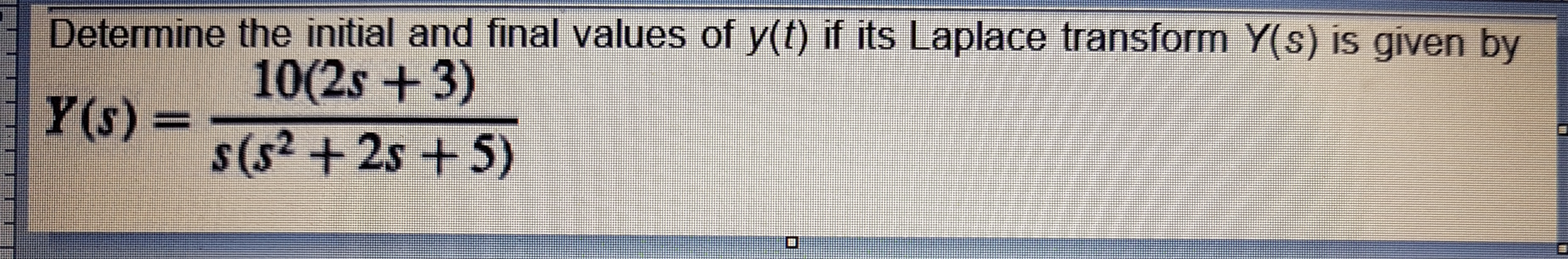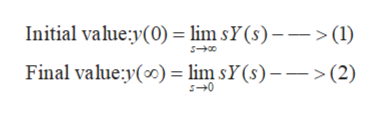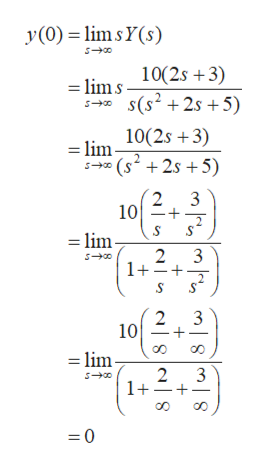# Determine the initial and final values of y(t) if its Laplace transform Y(s) is given by10(2s +3)Y(s)%3Ds(s2 +2s + 5)

Question
20 views

Solve the following problem:help_outlineImage TranscriptioncloseDetermine the initial and final values of y(t) if its Laplace transform Y(s) is given by 10(2s +3) Y(s)%3D s(s2 +2s + 5) fullscreen
check_circle

star
star
star
star
star
1 Rating
Step 1

According to the initial and final value theorem of Laplace transform, given a function Y(s), the initial and final value of the corresponding time domain function y(t) will be:help_outlineImage TranscriptioncloseInitial value:y(0) = lim sY(s) – –> (1) Final value:y(0) = lim sY(s) ––>(2) fullscreen
Step 2

In this case, the function Y(s) is given as

Step 3

So, using equation (1), the initial value of y(t) will be

...help_outlineImage Transcriptionclosey(0) = limsY(s) 10(2s +3) = lim s s* s(s² + 2s +5) 10(2s +3) = lim- (s² + 2s +5) 3 10 = lim- 3 1+=+ 10 = lim- 3 1+- fullscreen

### Want to see the full answer?

See Solution

#### Want to see this answer and more?

Solutions are written by subject experts who are available 24/7. Questions are typically answered within 1 hour.*

See Solution
*Response times may vary by subject and question.
Tagged in

### Electrical Engineering